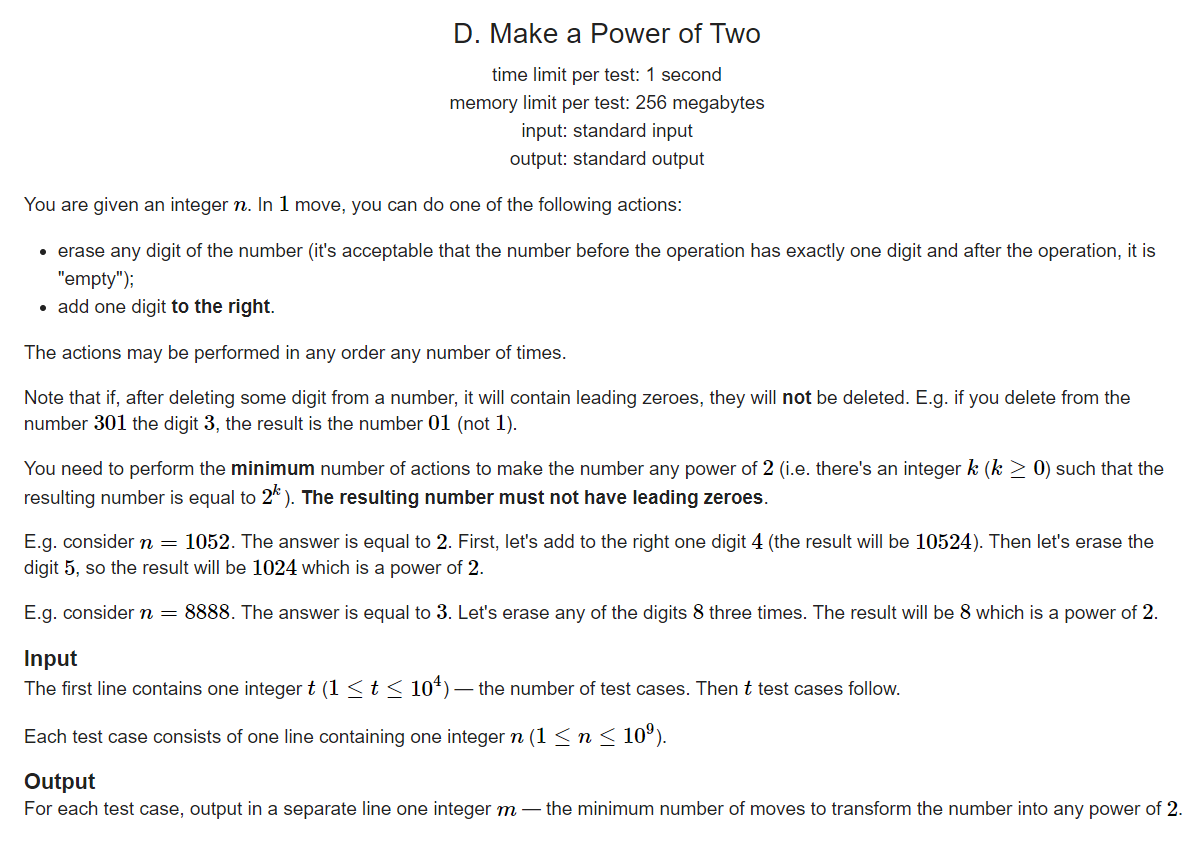# CF739 div3 D题 Make a Power of Two（打卡1）

2021/8/21 15:25:19 浏览：``````#include<iostream>
#include<string.h>
#include<cmath>
#include<sstream>
#include<algorithm>
#include<vector>
using namespace std;
vector<string> ts;
int main()
{
ios::sync_with_stdio(false);
for (long long i = 1; i < 1e18; i*=2)
{
ts.push_back(to_string(i));
//cout << i << endl;
}
cout << ts.size() << endl;
int T;
cin >> T;
string s;
while (T--)
{
cin >> s;
int p1 = 0, p2 = 0,r=2e6;
for (auto p : ts)
{
p2 = 0;
for (int i = 0; i < s.size(); i++)
if (s[i] == p[p2])
p2++;
r = min(r, (int)(p.size() - p2 + s.size() - p2));
}
cout << r << endl;
}
}
``````